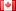# Revision history [back]

### elliptic curves in quartic and standard form

y^2 = ax^4+bx^3+cx^2+dx+e is birationally equivalent to an elliptic curve in standard Weierstrass form y^2=cubic(x). How to I get sage to exhibit/find the birational transformation that accomplishes that?

Warren D Smith warren.wds AT gmail.com2 No.2 RevisionDSM5232 ●21 ●68 ●112

### elliptic curves in quartic and standard form

y^2 = ax^4+bx^3+cx^2+dx+e
a*x^4+b*x^3+c*x^2+d*x+e


is birationally equivalent to an elliptic curve in standard Weierstrass form y^2=cubic(x). y^2=cubic(x). How to I get sage to exhibit/find the birational transformation that accomplishes that?

Warren D Smith warren.wds AT gmail.com

### elliptic curves in quartic and standard form

y^2 = a*x^4+b*x^3+c*x^2+d*x+e


is birationally equivalent to an elliptic curve in standard Weierstrass form y^2=cubic(x). How to I get sage to exhibit/find the birational transformation that accomplishes that?

[I am specifically interested in knowing all about this class of curves: 8Dy^2 = (x-2)(x-1)(x+1)*(x+2) for integer D. I am pretty new to both SAGE and elliptic curves.]4 eq formattingDSM5232 ●21 ●68 ●112

### elliptic curves in quartic and standard form

y^2 = a*x^4+b*x^3+c*x^2+d*x+e


is birationally equivalent to an elliptic curve in standard Weierstrass form y^2=cubic(x). How to I get sage to exhibit/find the birational transformation that accomplishes that?

[I am specifically interested in knowing all about this class of curves: 8Dy^2 8*D*y^2 = (x-2)(x-1)(x+1)*(x+2) (x-2)*(x-1)*(x+1)*(x+2) for integer D. I am pretty new to both SAGE and elliptic curves.]5 retaggedFrédéricC4131 ●3 ●37 ●85

### elliptic curves in quartic and standard form

y^2 = a*x^4+b*x^3+c*x^2+d*x+e


is birationally equivalent to an elliptic curve in standard Weierstrass form y^2=cubic(x). How to I get sage to exhibit/find the birational transformation that accomplishes that?

[I am specifically interested in knowing all about this class of curves: 8*D*y^2 = (x-2)*(x-1)*(x+1)*(x+2) for integer D. I am pretty new to both SAGE and elliptic curves.]